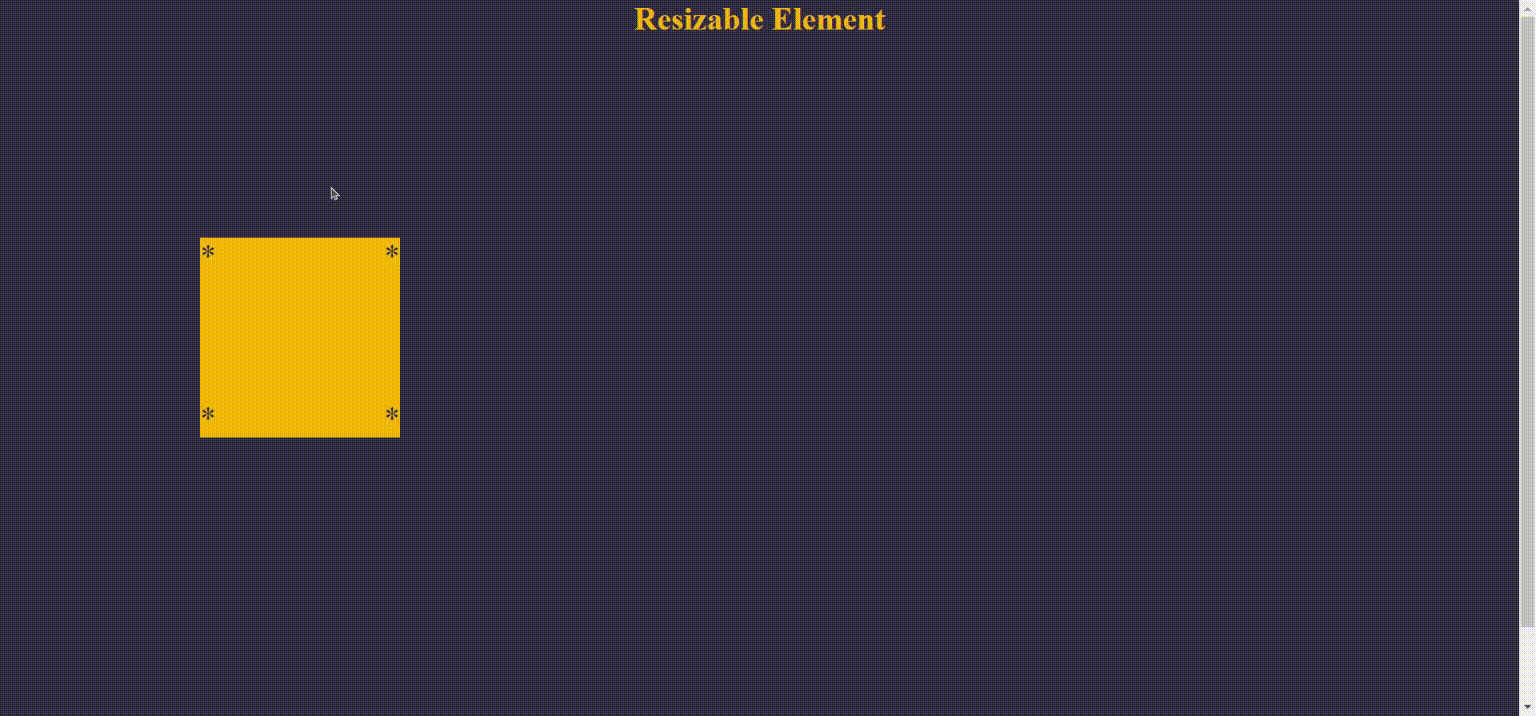# Largest Square of 1's- Dynamic Programming Solution

## Approach：

`def maximalSquare(self, matrix: List[List[str]]) -> int:        if not matrix:            return 0        nrows = len(matrix)        ncols = len(matrix)        max_square_len = 0        dp = [ * (ncols + 1) for i in range(nrows + 1)]        for i in range(1, nrows + 1):            for j in range(1, ncols + 1):                if (matrix[i - 1][j - 1] == '1'):                    dp[i][j] = min(dp[i][j - 1], dp[i - 1][j],                     dp[i    - 1][j - 1]) + 1                    max_square_len = max(max_square_len, dp[i][j])        return max_square_len ** 2`
`var maximalSquare = function(matrix) {    let copy = [...matrix]    let result = 0        for(let i = 0; i < copy.length; i++){        for (let j = 0; j < copy[i].length; j++){            if(i == 0 || j == 0 ){                copy[i][j]=matrix[i][j]            }else if(matrix[i][j]>0){                copy[i][j] = 1 + Math.min(copy[i][j-1], copy[i-1]                 [j], copy[i-1][j-1])            }            if(copy[i][j]> result){               result = copy[i][j]            }        }    }    return result ** 2};`

--

--

--

## More from chefvivica

Full-Stack Web Developer, Flatiron School Grad

Love podcasts or audiobooks? Learn on the go with our new app.

## How to Secure Your Express Application## React Native: Creating a Customized Drawer Navigator## Going to real life with Angular CLI## Building a Color Generator## Things that made me like Angular## 10 JavaScript Hacks Every Programmer Should Know## Create a resizeable element using Interact JS## chefvivica

Full-Stack Web Developer, Flatiron School Grad

## Corona is a Cross-Platform technology. What are the limits?## Is it so simple to promote a crypto project?## Legal Aspects of Blockchain Technology for Industrial Use Cases